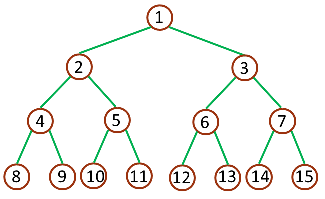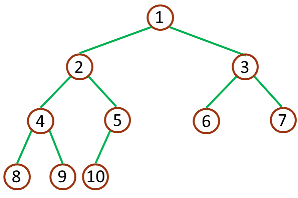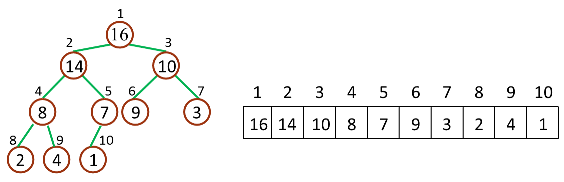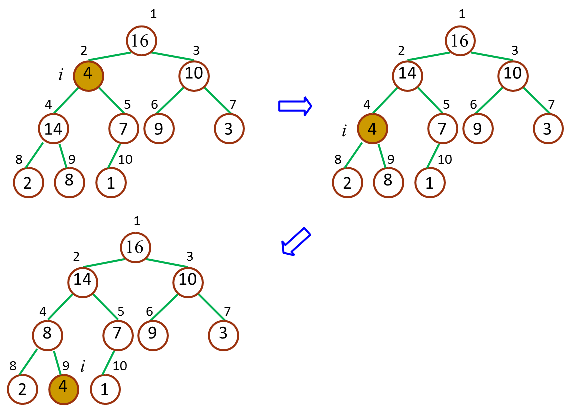• Parent(i) = floor(i/2)，i 的父节点下标
• Left(i) = 2i，i 的左子节点下标
• Right(i) = 2i + 1，i 的右子节点下标

Parent(i) = floor((i-1)/2)，i 的父节点下标
Left(i) = 2i + 1，i 的左子节点下标
Right(i) = 2(i + 1)，i 的右子节点下标